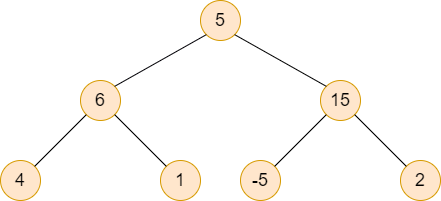New update is available. Click here to update.

# Path Sum ll

Last Updated: 26 Mar, 2021
Difficulty: Moderate

## PROBLEM STATEMENT

#### For Example:``````Consider the above binary tree. If ‘K’ is 15 then the required paths are [5, 6, 4] and [5, 15, -5].
``````
##### Input Format :
``````The first line contains an integer 'T' which denotes the number of test cases or queries to be run. Then the test cases are as follows.

The first line of each test case contains an integer ‘K’ which represents the number ‘K’.

The second line of each test case contains elements of the tree in the level order form. The line consists of values of nodes separated by a single space. In case a node is null, we take -1 in its place.

For example, the input for the tree depicted in the below image would be :
````````````1
2 3
4 -1 5 6
-1 7 -1 -1 -1 -1
-1 -1
``````

#### Explanation :

``````Level 1 :
The root node of the tree is 1

Level 2 :
Left child of 1 = 2
Right child of 1 = 3

Level 3 :
Left child of 2 = 4
Right child of 2 = null (-1)
Left child of 3 = 5
Right child of 3 = 6

Level 4 :
Left child of 4 = null (-1)
Right child of 4 = 7
Left child of 5 = null (-1)
Right child of 5 = null (-1)
Left child of 6 = null (-1)
Right child of 6 = null (-1)

Level 5 :
Left child of 7 = null (-1)
Right child of 7 = null (-1)

The first not-null node(of the previous level) is treated as the parent of the first two nodes of the current level. The second not-null node (of the previous level) is treated as the parent node for the next two nodes of the current level and so on.
The input ends when all nodes at the last level are null(-1).
``````
##### Note :
``````The above format was just to provide clarity on how the input is formed for a given tree.

The sequence will be put together in a single line separated by a single space. Hence, for the above-depicted tree, the input will be given as:

1 2 3 4 -1 5 6 -1 7 -1 -1 -1 -1 -1 -1
``````
##### Output Format:
``````For each test case, return all the paths that start from the root and end at the leaf and whose sum is exactly equal to ‘K’. All the paths should be printed in a separate line and should be enclosed in a square bracket.

Output for every test case will be printed in a separate line.
``````
##### Note:
``````You don’t need to print anything; It has already been taken care of. Just implement the given function.
``````
##### Constraints:
``````1 <= T <= 10
1 <= N <= 10^3
-10^5 <= K <= 10^5
-10^5 <= DATA <= 10^4

Time limit: 1 sec
``````## Approach 1

The basic idea is to perform Depth First Search on the given binary Tree and keep track of the current sum and whenever reached the lead node check if the sum becomes equal to the ‘K’ then pick this path, otherwise just backtrack to the previous node and exclude the value of this leaf node from the current sum.

We are here using a helper function that is recursive in nature and is used to find all the required paths.

``void helper(TreeNode<int> *root, vector<vector<int>> &reqPaths, int K, vector<int> curPath)``

Where “ROOT” is the root node of the given binary tree, “REQ_PATHS” is the vector that holds all the required paths, ‘K’ is the number described in the question, and “CUR_PATH” is the vector that holds all the nodes which involved in the current path.

The steps are as follows:

1. In the “HELPER” function
• If “ROOT” is NULL then return.
• Add the value of the current node in “CUR_PATH”.
• Check if this is the leaf node and its value is equal to the ‘K’ then push “CUR_PATH” in “REQ_PATHS”.
• Call for the left subtree with arguments as “ROOT”, “REQ_PATHS”, ‘K’ - the value of the current node, and “CUR_PATH”.
• Similarly, make a call for the right subtree.
• Remove the value of the current node from the “CUR_PATH”.
2. In the given function
• Create a vector of vectors “REQ_PATHS” that holds all the required Paths.
• Check if the “ROOT” is NULL then return “REQ_PATHS”.
• Create a vector “CUR_PATH” that will be used to store the value of nodes that are involved in the current path.
• Call the “HELPER” function with arguments as “ROOT’, “REQ_PATHS”, ‘K’, and ‘’CUR_PATH”.
• Return “REQ_PATHS”.